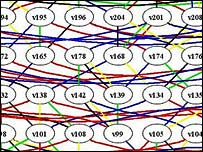A# Lie groupPart of the E8 matrix. Credit: David Vogan, MIT .

A Lie group is a group that is also a manifold. Lie groups were first described in the nineteenth century by the Norwegian mathematician Sophus Lie (pronounced 'Lee'). Lie groups of real matrices, such as occur in quantum field theory, give naturally occurring examples of Lie groups. The tangent space at the identity element of a Lie group forms a Lie algebra in a natural way.

In layperson's terms, a Lie group is a way of describing symmetrical objects. For example, there are Lie groups to describe the symmetry of simple objects such as balls, cylinders, cones. The most complicated Lie group that exists and, therefore, the most complicated symmetry known is a 248-dimensional structure called E8.

## E8

The Lie group known as E8 was discovered in 1887 but fully mapped only in 2007 after several years of effort by a team of 18 mathematicians. The final expression of it consisted of a 453,060 × 453,060 matrix. Each of the 205,263,363,600 entries in this matrix consists of equations, some of which are quite intricate.

E8 is much more than a mathematical curiosity, however. Physicists have encountered it increasingly in their efforts to unify gravity with the other fundamental forces in nature through theories such as string theory. The mapping of E8 may thus play a significant role in developing a consistent theory of quantum gravity.